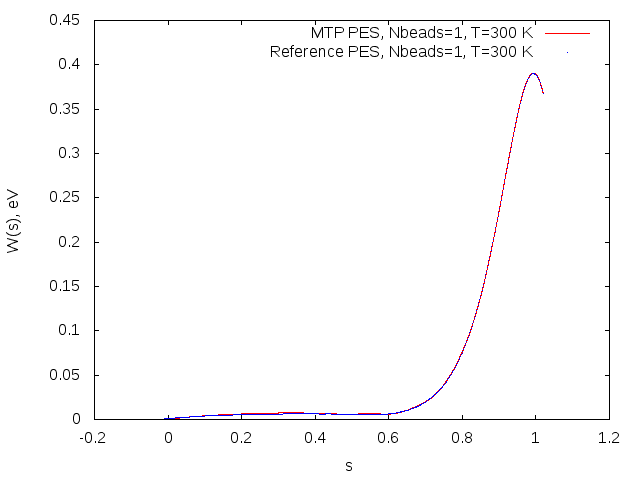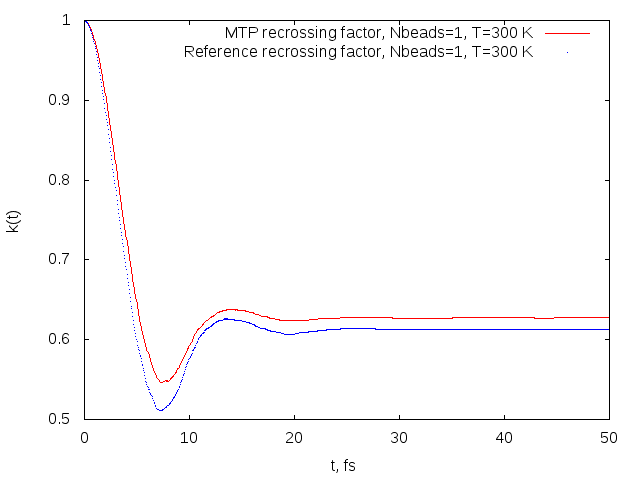# Prediction of chemical reaction rates using Machine Learning

The accurate and efficient calculation of chemical reaction rate coefficients is an important problem in computational chemistry. One of the methods allowing to resolve this problem is ring polymer molecular dynamics (RPMD, http://rpmdrate.cyi.ac.cy/) model which is based on the isomorphism between the quantum statistical mechanics of the physical system and the classical statistical mechanics of a fictitious ring polymer consisting of n copies of the system connected by harmonic springs. The resulting RPMD reaction rate theory is essentially a classical rate theory in an extended (discretized imaginary time path integral) phase space.

RPMD reaction rate theory has been demonstrated to be very accurate in prediction the rates of various chemical reactions in wide temperature ranges (e.g., OH+H2→H+H2O in 150-2000K, CN+CH4→HCN+CH3 in 300-1500K). Nevertheless, application of RPMD for the rate coefficients predicition requires efficient (i.e., accurate and fast) interatomic interaction models. For many reactions that are important, e.g. for astrochemistry, there is no accurate interatomic interaction model whereas quantum mechanical calculations that are accurate enough are too time consuming.

This project is aimed to prediction of chemical reactions rate coefficients using machine learning. Namely, on the basis of our Moment Tensor Potential (MTP) and active learning methodology developed in our group we effectively select atomic configurations obtained during RPMD, learn MTP on these configurations and predict the rate coefficients with high accuracy. The graphics with the potential mean force profiles and the transmission coefficients that are necessary to calculate the rate constants and the comparison of the rate coefficients are shown below.Potential of mean force profiles obtained on MTP (red) and reference model (blue) for the OH+H2->H2O+H reaction.Recrossing factors obtained on MTP (red) and reference model (blue) for the OH+H2->H2O+H reaction.

MTP rate coefficient: 2.02*108 cm3/(mol*s)

Reference model rate coefficient: 2.12*108 cm3/(mol*s)